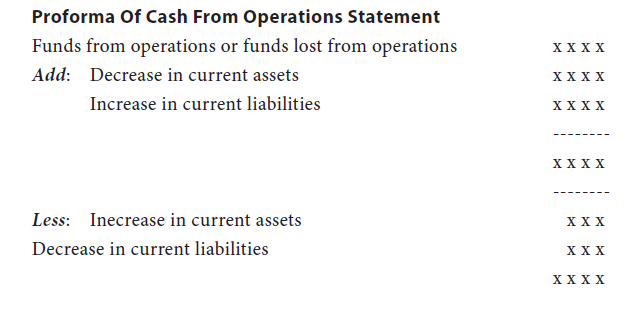Home | ARTS | Accounting For Managers | Calculation of Cash From Operations

# Calculation of Cash From Operations

Posted On :  25.02.2018 09:33 am

The important step in the preparation of cash flow statement is the calculation of cash from operations.

It is calculated as follows:
The first step in the calculation of cash from operations is the calculation of funds from operations (which is already explained in the lesson on funds flow analysis). To the funds from operations the decrease in current assets and increase in current liabilities will be added (except cash, bank and bank o.d.). From the added total, increase in current assets and decrease in current liabilities will be deducted (except cash, bank and bank o.d.). The resultant figure is cash from operationsCash from operations or cash lost from operations
As in the case of fund flow analysis here also we assume provision for taxation and proposed dividend as current liabilities.
Tags : Accounting For Managers - Funds Flow Analysis And Cash Flow Analysis
Last 30 days 730 views